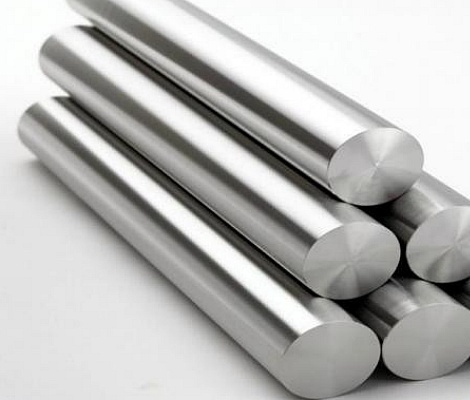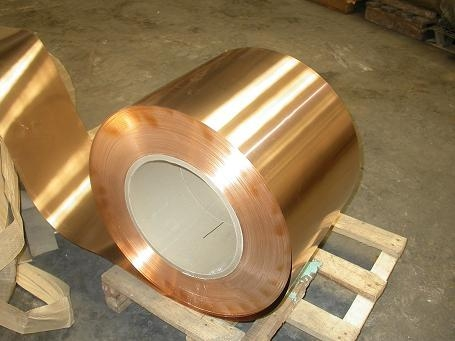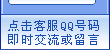局部/满涂绝缘 局部纳米散热 铍铜(C1720) 钛铜(C1990//YCUT) 高强度特殊铜 高导电铜合金 白铜 铍铜真空热处理 铬锆铜合金(C18150/18400) 五金冲压件
4新闻中心

### 常用金属材料计算公式 实用大报价常备• 圆钢重量（公斤）=0.00617×直径×直径×长度

• 方钢重量（公斤）=0.00785×边宽×边宽×长度

• 六角钢重量（公斤）=0.0068×对边宽×对边宽×长度

• 八角钢重量（公斤）=0.0065×对边宽×对边宽×长度

• 螺纹钢重量（公斤）=0.00617×计算直径×计算直径×长度

• 角钢重量（公斤）=0.00785×（边宽+边宽-边厚）×边厚×长度

• 扁钢重量（公斤）=0.00785×厚度×边宽×长度

• 钢管重量（公斤）=0.02466×壁厚×（外径-壁厚）×长度

• 钢板重量（公斤）=7.85×厚度×面积• 圆紫铜棒重量（公斤）=0.00698×直径×直径×长度

• 圆黄铜棒重量（公斤）=0.00668×直径×直径×长度

• 方紫铜棒重量（公斤）=0.0089×边宽×边宽×长度

• 方黄铜棒重量（公斤）=0.0085×边宽×边宽×长度

• 六角紫铜棒重量（公斤）=0.0077×对边宽×对边宽×长度

• 六角黄铜棒重量（公斤）=0.00736×边宽×对边宽×长度

• 紫铜板重量（公斤）=0.0089×厚×宽×长度

• 黄铜板重量（公斤）=0.0085×厚×宽×长度

• 圆紫铜管重量（公斤）=0.028×壁厚×（外径-壁厚）×长度

• 圆黄铜管重量（公斤）=0.0267×壁厚×（外径-壁厚）×长度• 圆铝棒重量（公斤）=0.0022×直径×直径×长度

• 方铝棒重量（公斤）=0.0028×边宽×边宽×长度

• 六角铝棒重量（公斤）=0.00242×对边宽×对边宽×长度

• 铝板重量（公斤）=0.00171×厚×宽×长度

• 园铝管重量（公斤）=0.00879×壁厚×（外径-壁厚）×长度

• 丝网的重量：丝直径x丝直径x目数=1平方米的（市斤）重量

• 钢板网的重量：1平方米钢板的重量÷延长率=1平方米钢板网的重量

• 钢板的重量：板厚x比重=1平方米钢板的重量

304不锈钢的密度

1Cr18Ni9，0Cr19Ni19，00Cr19Ni11， 0Cr18Ni11Ti，（1Cr18Ni9Ti） 7.93

0Cr25Ni20，0Cr17Ni12Mo2， 00Cr17Ni14Mo2，0Cr18Ni11Nb  7.98

00Cr17 7.70

0Cr13，00Cr18Mo2 7.75

Weight（kg）=Thickness（mm）*Width（m）*Length（m）*Density（g/cm3）

7.93           201,202,301,302,304,304L,305,321

7.98                 309S,310S,316,316L,347

7.75                          405,410,420

7.70                          409,430,434

304、321管每米重量(kg)计算公式:0.02491*壁厚(mm)*(外径-壁厚)(mm)

316L、310S管每米重量(kg)计算公式：0.02495*壁厚(mm)*(外径-壁厚)(mm)

W（重量，kg ）=F(断面积 mm2)×L(长度，m)×ρ(密度，g/cm3)×1/1000

W= 0.006165 ×d×d

d= 直径mm

W= 0.00617 ×d×d

d= 断面直径mm

W= 0.00785 ×a ×a

a= 边宽mm

W= 0.00785 ×b ×d

b= 边宽mm

d= 厚mm

W= 0.006798 ×s×s

s= 对边距离mm

W= 0.0065 ×s ×s

s= 对边距离mm

W = 0.00785 ×[d （2b – d ）+0.215 （R2 – 2r 2 ）]

b= 边宽

d= 边厚

R= 内弧半径

r= 端弧半径

W= 0.00785 ×[d （B+b – d ）+0.215 （R2 – 2 r 2 ）]

B= 长边宽

b= 短边宽

d= 边厚

R= 内弧半径

r= 端弧半径

W=0.00785 ×[hd+2t （b – d ）+0.349 （R2 – r 2 ）]

h= 高

b= 腿长

d= 腰厚

t= 平均腿厚

R= 内弧半径

r= 端弧半径

W= 0.00785 ×[hd+2t （b – d ）+0.615 （R2 – r 2 ）]

h= 高

b= 腿长

d= 腰厚

t= 平均腿厚

R= 内弧半径

r= 端弧半径

W= 7.85 ×d

d= 厚

W= 0.02466 ×S （D – S ）

D= 外径

S= 壁厚《中华人民共和国电信与信息服务业务经营许可证》编号：粤ICP备16117657号
*本站相关网页素材及相关资源均来源互联网，如有侵权请速告知，我们将会在24小时内删除* [后台管理]